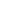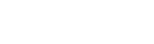## Articles## What is Wavespeed?The wavespeed is the propagation speed at which waves will be transmitted through a medium. When performing waterhammer analysis, the wavespeed through the fluid must be known to calculate how fast pressure waves will propagate through the pipes, as well as the severity of pressure changes due to changes in flow.

How is Wavespeed Calculated?

Several parameters are used for wavespeed calculations.

The acoustic velocity, or the velocity of the wave travelling uninterrupted through a medium, is the first factor. The acoustical velocity is calculated as follows:Where

K = fluid bulk modulus of elasticity

ρ = fluid density

The wavespeed in a piping system will be slower than the acoustic velocity as the pressure waves will be distorted by the restriction of the pipe walls, losing energy in the process of propagation. The effect of distortions in the pressure waves due to the pipes can be accounted for by modifying the acoustical velocity as follows:Where

E = pipe modulus of elasticity

D = pipe inner diameter

e = wall thickness

c1 = pipe support constant

The equations to calculate the pipe support constant (c1) can be found in Wylie & Streeter . The pipe support constant adjusts the wavespeed equation to accurately account for the pipe strains and stresses due to elongation of the material when different pipe supports are used. The pipe material poisson’s ratio and/or the pipe geometry are used to calculate c1, depending on what supports are used. The pipe support constant will be 1 for thin-walled pipe anchored throughout with expansion joints, and will be slightly higher or lower for other types of supports.

Often engineers do not know the exact composition of the pipe materials, so parameters such as the pipe modulus of elasticity and poisson’s ratio must be estimated. Thus calculations for the wavespeed inherently have a high level of uncertainty.

A Numerical Example

Consider a piping network with water at 72˚F (22˚C) and an average pressure of 290 psia (20 bar). From the NIST REFPROP fluid database the density is 62.3 lbm/ft3 (998 kg/m3) and the bulk modulus is about 3.2 E+05 psia (2.2 E+04 bar).

The water is flowing through a Steel-ANSI STD 18-inch pipe. The pipe schedule shows that the inside diameter is 17.25 inches (43.8 cm), and the wall thickness is 0.375 inches (0.952 cm). The modulus of elasticity found in Nayar’s Piping Handbook is about 2.9 E+07 psia (2.0 E+06 bar). The Poisson ratio is listed as 0.3 in Perry’s Chemical Engineer’s Handbook. Assuming the pipe is thick-walled anchored throughout, the pipe support constant c1 is 0.88.

By entering the above information into Equation 2, the wavespeed is approximately 4060 ft/sec.

As there is a high level of uncertainty in the wavespeed, a sensitivity analysis for wavespeed may be recommended depending on the calculation being performed. Conveniently, much of this information is standardized so waterhammer analysis software can determine wavespeed easily.

References

 Wylie, E. B. and Streeter, V. L., 1993, Fluid Transients in Systems, Prentice Hall, Englewood Cliffs, NJ.

What is Wavespeed?

The wavespeed is the propagation speed at which waves will be transmitted through a medium. When performing waterhammer analysis, the wavespeed through the fluid must be known to calculate how fast pressure waves will propagate through the pipes, as well as the severity of pressure changes due to changes in flow.

How is Wavespeed Calculated?

Several parameters are used for wavespeed calculations.

The acoustic velocity, or the velocity of the wave travelling uninterrupted through a medium, is the first factor. The acoustical velocity is calculated as follows:

Where

K = fluid bulk modulus of elasticity

ρ = fluid density

The wavespeed in a piping system will be slower than the acoustic velocity as the pressure waves will be distorted by the restriction of the pipe walls, losing energy in the process of propagation. The effect of distortions in the pressure waves due to the pipes can be accounted for by modifying the acoustical velocity as follows:

Where

E = pipe modulus of elasticity

D = pipe inner diameter

e = wall thickness

c1 = pipe support constant

The equations to calculate the pipe support constant (c1) can be found in Wylie & Streeter . The pipe support constant adjusts the wavespeed equation to accurately account for the pipe strains and stresses due to elongation of the material when different pipe supports are used. The pipe material poisson’s ratio and/or the pipe geometry are used to calculate c1, depending on what supports are used. The pipe support constant will be 1 for thin-walled pipe anchored throughout with expansion joints, and will be slightly higher or lower for other types of supports.

Often engineers do not know the exact composition of the pipe materials, so parameters such as the pipe modulus of elasticity and poisson’s ratio must be estimated. Thus calculations for the wavespeed inherently have a high level of uncertainty.

A Numerical Example

Consider a piping network with water at 72˚F (22˚C) and an average pressure of 290 psia (20 bar). From the NIST REFPROP fluid database the density is 62.3 lbm/ft3 (998 kg/m3) and the bulk modulus is about 3.2 E+05 psia (2.2 E+04 bar).

The water is flowing through a Steel-ANSI STD 18-inch pipe. The pipe schedule shows that the inside diameter is 17.25 inches (43.8 cm), and the wall thickness is 0.375 inches (0.952 cm). The modulus of elasticity found in Nayar’s Piping Handbook is about 2.9 E+07 psia (2.0 E+06 bar). The Poisson ratio is listed as 0.3 in Perry’s Chemical Engineer’s Handbook. Assuming the pipe is thick-walled anchored throughout, the pipe support constant c1 is 0.88.

By entering the above information into Equation 2, the wavespeed is approximately 4060 ft/sec.

As there is a high level of uncertainty in the wavespeed, a sensitivity analysis for wavespeed may be recommended depending on the calculation being performed. Conveniently, much of this information is standardized so waterhammer analysis software can determine wavespeed easily.

References

 Wylie, E. B. and Streeter, V. L., 1993, Fluid Transients in Systems, Prentice Hall, Englewood Cliffs, NJ.

### Category

##### Your resource to prevent pressure transients in piping systems caused by waterhammer. This page is owned by Applied Flow Technology. Collaborators are always welcome.
© Copyright 2021 Applied Flow Technology. All Rights Reserved.